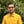Trusted answers to developer questions
Trusted Answers to Developer Questions

Related Tags

dart
communitycreator

# What is max() in Dart?Harris Amjad

Grokking Modern System Design Interview for Engineers & Managers

Ace your System Design Interview and take your career to the next level. Learn to handle the design of applications like Netflix, Quora, Facebook, Uber, and many more in a 45-min interview. Learn the RESHADED framework for architecting web-scale applications by determining requirements, constraints, and assumptions before diving into a step-by-step design process.

The max() function returns the largest value of two numbers sent as parameters.

The figure below shows a visual representation of the max() function.

Visual representation of the max() function

To use the max() function, you will need to import the dart:math library as shown below:

import dart:math


### Syntax

The syntax of the max() function is as follows:

type max(num-1, type num-2)
// type extends number


### Parameters

The max() function takes two numbers as parameters.

The numbers can be of type Int, Double, Float, or Long.

### Return value

The max() function returns the largest value from the two numbers sent as parameters.

### Code

The code below shows how to use max() in Dart.

import 'dart:convert';import 'dart:math';void main() {  // two positive numbers  print("The value of max(10, 0) = ${max(10, 0)}"); //one positive and the other negative print("The value of max(4, -6) =${max(4, -6)}");   // both negative numbers  print("The value of max(-10, -9) = ${max(-10, -9)}"); // both double numbers print("The value of max(12.234,1.2345) =${max(12.234,1.2345)}");  // one int and the other double   print("The value of max(12.234,1) = \${max(12.234,1)}");}

For every pair of values in the code above, the max() function returns the larger of the two.

RELATED TAGS

dart
communitycreator

CONTRIBUTORHarris Amjad

Grokking Modern System Design Interview for Engineers & Managers

Ace your System Design Interview and take your career to the next level. Learn to handle the design of applications like Netflix, Quora, Facebook, Uber, and many more in a 45-min interview. Learn the RESHADED framework for architecting web-scale applications by determining requirements, constraints, and assumptions before diving into a step-by-step design process.

Keep Exploring

Learn in-demand tech skills in half the time